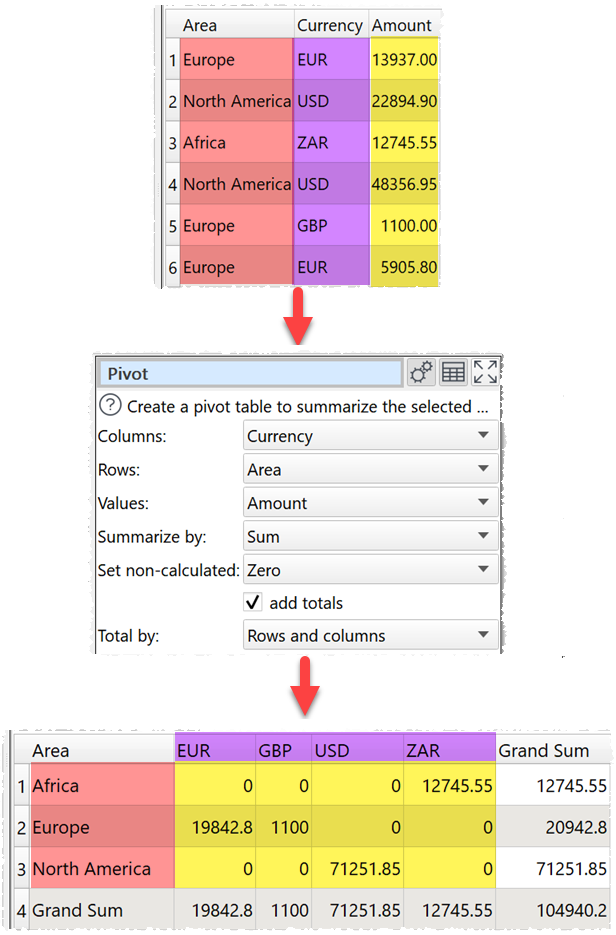﻿ Reference > Transforms > Pivot

# Pivot

## Description

Creates a pivot table to summarize values for one or two columns.

## Example

Pivot to sum 'Amount' by 'Area' and 'Currency':One.

## Options

Set Columns to the column values you want to use as columns in your pivot table.

Set Rows to the column values you want to use as rows in your pivot table.

Set Values to the column you wish to summarize.

Set Summarize by to how you wish to summarize the values:

oSum show the sum of the values. Non-numeric and empty values are ignored.

oMinimum shows the smallest value. Non-numeric and empty values are ignored.

oMaximum shows the largest value. Non-numeric and empty values are ignored.

oAverage shows the arithmetic mean of the values. Non-numeric and empty values are ignored.

oMedian shows the median of numeric values in the column. Non-numeric and empty values are ignored.

oMode shows the mode of numeric values in the column. Non-numeric and empty values are ignored.

oStandard deviation is the sample standard deviation (equivalent to Excel function stddev.s). Non-numeric and empty values are ignored.

oCount shows the number of non-empty values (a value that contains whitespace or 0 is not considered empty).

Set Set non-calculated depending on how you want to set cells not calculated by the pivot.

Set Total by depending on whether you want to total by Rows and columns, Rows or Columns. This is only available if add totals is checked and Columns and Rows are selected.

Check drilldown to allow double-clicking a cell in the data table to drilldown to the rows that contributed to this value in the upstream data.

## Notes

Column and row values are ordered alphabetically. You can change the order using Reorder Cols (for columns) and Sort (for rows).

Mode may not work as expected with non-integer values, due to precision issues. You can fix this by using a Num Format transform first.

Use Num Format to change the precision of the results.

Use Scale to convert the results to percentages.

To add a column of 1 values to Sum (e.g. to find out how many rows a value occurs in) use a New Col transform.